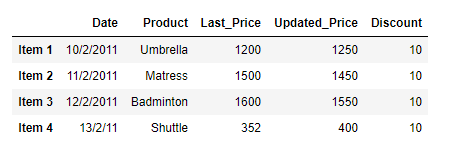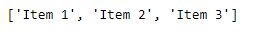# Return the Index label if some condition is satisfied over a column in Pandas Dataframe

• Last Updated : 26 Jan, 2019

Given a Dataframe, return all those index labels for which some condition is satisfied over a specific column.

Solution #1: We can use simple indexing operation to select all those values in the column which satisfies the given condition.

 `# importing pandas as pd``import` `pandas as pd`` ` `# Create the dataframe``df ``=` `pd.DataFrame({``'Date'``:[``'10/2/2011'``, ``'11/2/2011'``, ``'12/2/2011'``, ``'13/2/2011'``],``                   ``'Product'``:[``'Umbrella'``, ``'Matress'``, ``'Badminton'``, ``'Shuttle'``],``                   ``'Last_Price'``:[``1200``, ``1500``, ``1600``, ``352``],``                   ``'Updated_Price'``:[``1250``, ``1450``, ``1550``, ``400``],``                   ``'Discount'``:[``10``, ``10``, ``10``, ``10``]})`` ` `# Create the indexes``df.index ``=``[``'Item 1'``, ``'Item 2'``, ``'Item 3'``, ``'Item 4'``]`` ` `# Print the dataframe``print``(df)`

Output :Now, we want to find out the index labels of all items whose ‘Updated_Price’ is greater than 1000.

 `# Select all the rows which satisfies the criteria``# convert the collection of index labels to list.``Index_label ``=` `df[df[``'Updated Price'``]>``1000``].index.tolist()`` ` `# Print all the labels``print``(Index_label)`

Output :As we can see in the output, the above operation has successfully evaluated all the values and has returned a list containing the index labels.

Solution #2: We can use Pandas `Dataframe.query()` function to select all the rows which satisfies some condition over a given column.

 `# importing pandas as pd``import` `pandas as pd`` ` `# Create the dataframe``df ``=` `pd.DataFrame({``'Date'``:[``'10/2/2011'``, ``'11/2/2011'``, ``'12/2/2011'``, ``'13/2/2011'``],``                   ``'Product'``:[``'Umbrella'``, ``'Matress'``, ``'Badminton'``, ``'Shuttle'``],``                   ``'Last_Price'``:[``1200``, ``1500``, ``1600``, ``352``],``                   ``'Updated_Price'``:[``1250``, ``1450``, ``1550``, ``400``],``                   ``'Discount'``:[``10``, ``10``, ``10``, ``10``]})`` ` `# Create the indexes``df.index ``=``[``'Item 1'``, ``'Item 2'``, ``'Item 3'``, ``'Item 4'``]`` ` `# Print the dataframe``print``(df)`

Output :Now, we want to find out the index labels of all items whose ‘Updated_Price’ is greater than 1000.

 `# Select all the rows which satisfies the criteria``# convert the collection of index labels to list.``Index_label ``=` `df.query(``'Updated_Price > 1000'``).index.tolist()`` ` `# Print all the labels``print``(Index_label)`

Output :My Personal Notes arrow_drop_up